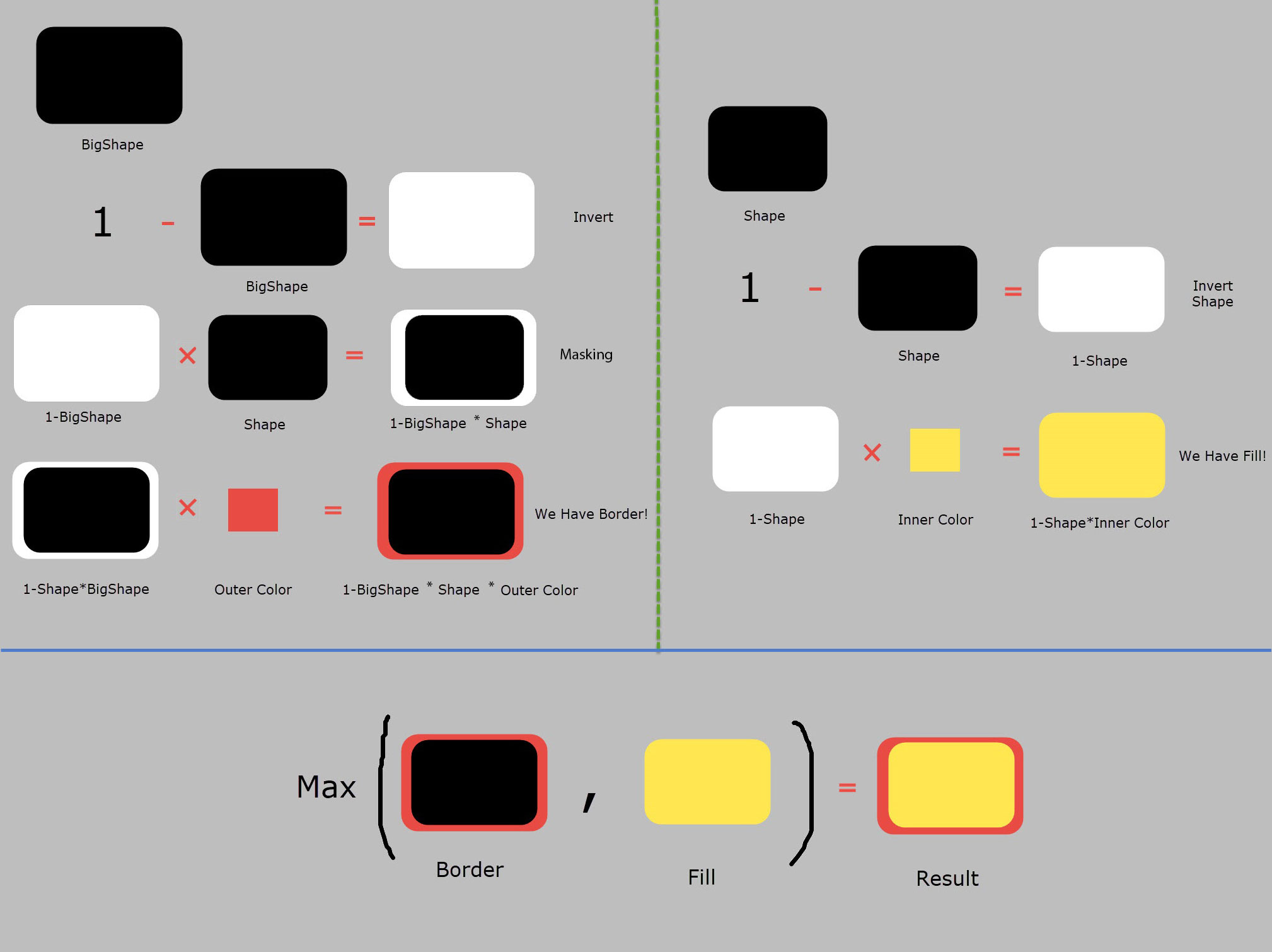# GLSL - Overlap shapes generated by the same function with custom size/color

I'm trying to make a ui button shader by overlaping a rounded rectangle on another rounded rectangle but with different size and gradient color. This way I'll have a small outline of a color (e.g. grey) and the body with a grey-ish gradient.

This is how my fragment code looks so far:

#ifdef GL_ES
precision mediump float;
#endif

uniform vec2 u_resolution;

const float PI = 3.14159265;

// from http://www.iquilezles.org/www/articles/distfunctions/distfunctions.htm
float rect( vec2 p, vec2 size, float r ) {
return length(max(abs(p)-size+r,0.0))-r;
}

void main() {
vec2 halfRes = 0.5 * u_resolution.xy;
float aspect = u_resolution.y/u_resolution.x;
// compute box
float b = rect(gl_FragCoord.xy - halfRes, halfRes, 30.0);
b *= rect(gl_FragCoord.xy - halfRes, halfRes * vec2(0.99, 0.96), 30.0);

// colorize (red / black )
vec4 c = mix( vec4(0.326,0.603,1.000,1.000), vec4(0.0), smoothstep(0.0, 13.264,b) );

gl_FragColor = c;
}


You can modify it directly here by cp http://editor.thebookofshaders.com/

EDIT: I've managed to get the border, now I'll only need to fill the hollow..

• So, what's the problem? – Bálint Dec 23 '17 at 18:45
• I can't figure it out to draw another small shape into it since I'm using mix(). I've tried substracting, adding, multipling but I don't think I understood mix corectly.. – alex1997 Dec 23 '17 at 18:52
• The rect function returns a distance from the rectangle, why not simply check if the distance from the inner rectangke is 0, then color the pixel to that color, if it's not but the distance from the outer rectangle is 0, then color the pixel to that color – Bálint Dec 23 '17 at 19:03
• This sounds like step() function, but it's not gonna solve me – alex1997 Dec 23 '17 at 19:05

now you can control inner and outer color for UI button. if you need more option like gradiant this is useful link: https://thebookofshaders.com/06/#ifdef GL_ES
precision mediump float;
#endif

uniform vec2 u_resolution;

vec2 scale = vec2(0.85,0.6);
vec2 transform = vec2(-50.,-90.);

vec4 rect( vec2 p, vec2 size, float r ,vec4 InnerColor,vec4 OuterColor) {

float shape = length(max(abs(p)-size+r,0.0))-r;
float bigshape = length(max(abs(p)-(size+9.)+r,0.0))-r;
vec4 border = (1.-bigshape*shape)*floor(OuterColor);
vec4 fill = (1.-shape)*floor(InnerColor);
return max(border,fill );
}

void main() {
vec2 halfRes = 0.5 * u_resolution.xy;
halfRes *= scale;

float aspect = u_resolution.y/u_resolution.x;
aspect = aspect;
// compute box
vec4 Rectangle = rect(gl_FragCoord.xy+transform - halfRes, halfRes, 80.,vec4(1.,1.,0.,1.),vec4(1.,0.,0.,1.));

gl_FragColor = vec4(Rectangle);
}

• Looks good, but it's a bit hardcoded, I'll see if I can fixt it, but it shouldn't be hard – alex1997 Dec 23 '17 at 22:53
• @alex1997 I edited my question and explain it in the picture :D – Seyed Morteza Kamali Dec 24 '17 at 4:49
• Thanks man! I finally got what I wanted, but moreover, I learnt do it by myself! – alex1997 Dec 24 '17 at 13:21